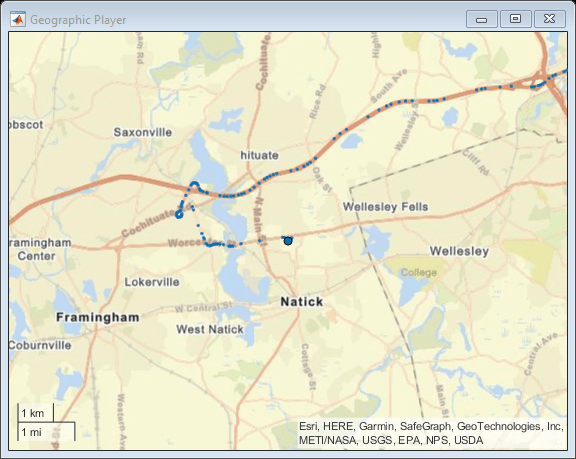Documentation

### This is machine translation

Mouseover text to see original. Click the button below to return to the English version of the page.

# isOpen

Return true if `geoplayer` figure is visible

## Syntax

``tf = isOpen(player)``

## Description

example

````tf = isOpen(player)` returns logical `1` (true) if the `geoplayer` figure is visible. Otherwise, `isOpen` returns logical `0` (false).```

## Examples

collapse all

Load a sequence of latitude and longitude coordinates.

`data = load('geoRoute.mat');`

Create a geographic player with a zoom level of 12. Configure the player to display all points in its history.

`player = geoplayer(data.latitude(1),data.longitude(1),12,'HistoryDepth',Inf);`

Display the geographic coordinates in a sequence by using the `plotPosition` function. Put the call to `plotPosition` inside a `while` loop, so that the player plots points only while the figure is open. You can exit the loop by closing the figure. If you do not close the figure, then the loop automatically exits when all points are plotted.

```i = 1; numPoints = length(data.latitude); while isOpen(player) && i<=numPoints plotPosition(player,data.latitude(i),data.longitude(i)) pause(0.1) i=i+1; end```

To make the figure visible again, use the `show` function.

`show(player)`## Input Arguments

collapse all

Streaming geographic player, specified as a `geoplayer` object.

## Output Arguments

collapse all

Visibility of geographic player, returned as logical `1` (true) when the `geoplayer` figure is open, and logical `0` (false) otherwise.